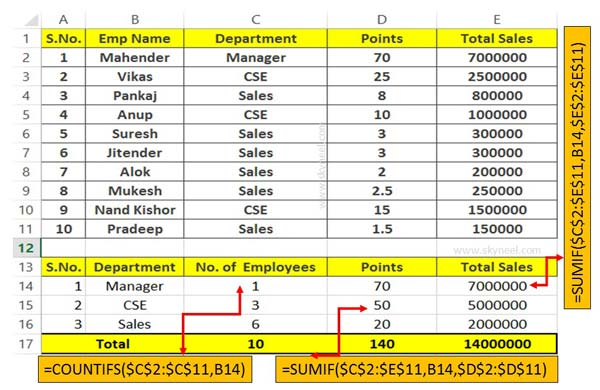# How to use COUNTIFS and SUMIF together in Excel

COUNTIFS and SUMIF important functions of Excel. If you have complex criteria then you can use these functions to get the result in Worksheet

If you want to become an Excel experts then you must have complete command on Excel functions and different options. Sometime you need to use countifs and sumif function together in Excel sheet to count and sum the resulted values.

### Countifs function in Excel

Countifs is an important function of Excel. This function located in the Statistical function category. It allows you to quickly count number of cells specified by a given set of more than one conditions or criteria.

This function count the number of cells within the given range of cells or any array that match the multiple condition.

Syntax of Countifs: COUNTIFS(range1, criteria1,range2, criteria2…)

Range1, range2, … : You can give up to 1 to 127 ranges which include any number, name, array or reference that contain any kind of number, but blank and text values are ignored.

Criteria1, criteria2, … You can give up to 1 to 127 conditions in which you can set any number, expression, cell address, or any text value that define which cells will be counted.

### Sumif function in Excel

SUM is an important function used to get the total of selected range of cells. But sometime we need to sum selected range of cells which match the given condition. In that situation you have to use SUMIF function at the place of SUM function in Excel. SUMIF is an important function of Excel which is located in the Mathematical and Trig category. This function is used to conditionally sum the specified range values which match the given condition.

Syntax of Sumif: SUMIF(range, criteria, [sum_range])

range: It the range of continuous cells that you want to evaluated by the given criteria.

criteria: It is a condition which specifies that which items you want to add that match the given criteria in the range.

sum_range: It is an optional argument which specifies which cells to be added. If “sum_range” argument is omitted then “range” is used as a “sum_range”.

## How to use COUNTIFS and SUMIF together in Excel

Excel has lot of inbuilt functions which you can used to solve complex criteria. If you have one or more criteria then you have to use function to get the correct result.For eg: If you have an employee worksheet data and want to count how much number of employees working in specific department. Along with you want to get total points and total sales amount as per the specific department. In that situation you must have to use Countifs and Sumif function in your active worksheet.

Step 1: Prepare the following worksheet to use Countifs and Sumif functions together in Excel sheet.

Step 2: If you want to count number of employees who are working in specific department then use given formula in cell C14. =COUNTIFS(\$C\$2:\$C\$11,B14) and press enter. Now you can drag the formula with the help of fill handle. You can also copy the formula using Excel shortcut keys from C14 to C16.

Step 3: To get the total points of employees which match the given department as a criteria you have to apply sumif function in cell D14 =SUMIF(\$C\$2:\$E\$11,B14,\$D\$2:\$D\$11). Now you can drag or copy the formula from D14:D16.

Step 4: To calculate total sales of employees use sumif function in cell E14 =SUMIF(\$C\$2:\$E\$11,B14,\$E\$2:\$E\$11). Now copy the given formula from rest of the cells.

Note: To copy the formula use F4 key to change the reference type from relative to absolute reference.

It is an quick guide which helps you to using Countifs and Sumif together in Excel sheet. If you have any complicated criteria then you can use different Excel functions as per your need.